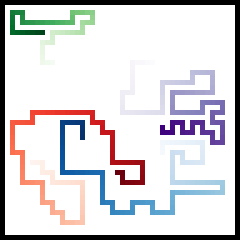QR codes are quite interesting, but boring to look at. For this reason I introduce SR codes, which stands for Snake Race codes. It improves QR codes on several fronts:

• Animation!
• Color!
• Hardcore. QR codes have error correction. SR codes don't. If you make an error it's game over.
• Not quick. Savour the wait before you can scan the code.

Developing a scanner app is left as an exercise for the reader :)QR codes are really interesting. Error correction and packing as much information as you can in a small amount of pixels while keeping it robust is a nice challenge.

Nice explanation in Dutch https://michielp1807.github.io/qr-codes/ or check out the Wikipedia page.

## Representing position and orientation

We represent the position of the snake as a point $(x, y)$ with an orientation $(h, v)$. Taking turns is done with a rotation matrix that defines 90 degree turns and going straight (=not changing direction).

actions = [
# Left
np.array([
[0, 1],
[-1, 0]
]),
# Straight
np.array([
[1, 0],
[0, 1]
]),
# Right
np.array([
[0, -1],
[1, 0]
])
]


For example, if the snake is going to the right it has orientation $(1,0)$. If it makes a 90 degree turn to the left it goes up and has orientation $(0, -1)$. The first coordinate is horizontal and the second is vertical.

actions @ np.array([1, 0])

array([ 0, -1])

Going left four times we should end up in the same direction:

orientation = np.array([1, 0])
for i in range(4):
orientation = actions @ orientation
orientation

array([1, 0])

## Going places

We want to see where the snakes have been as well as their current position. Below we define:

• The different snakes in snakes, including start position, color and trail
• For each snake we also keep track if they are active. The snake stops if there is no valid move to make.
• To make our lives easy we have per position the information if some snake has been there to limit possible moves matrix

Define a method to generat the trails of the snakes

def generate_trails(size: int, bits):
# Define quarter distance
q = size // 4

# Define the start positions of all snakes
snakes = [
{
'c': 'Greens',
'position': np.array([q+1, q+1]),
'orientation':  np.array([1, 0]),
'trail': np.full((2*(size+2), 2*(size+2)), 0),
'active': True
},
{
'c': 'Reds',
'position': np.array([3*q+1, 1+1]),
'orientation': np.array([0, 1]),
'trail': np.full((2*(size+2), 2*(size+2)), 0),
'active': True
},
{
'c': 'Blues',
'position': np.array([3*q+1, 3*q+1]),
'orientation': np.array([-1, 0]),
'trail': np.full((2*(size+2), 2*(size+2)), 0),
'active': True
},
{
'c': 'Purples',
'position': np.array([q+1, 3*q+1]),
'orientation': np.array([0, -1]),
'trail': np.full((2*(size+2), 2*(size+2)), 0),
'active': True
}
]

# Keep track of where the snakes have passed
matrix = np.full((size+2, size+2), False)

# Set the borders
matrix[0, :] = True
matrix[:, 0] = True
matrix[-1, :] = True
matrix[:, -1] = True

# Go over the bits
current_bit = 0
iteration = 0

# While there are active snakes try it out
while any([snake['active'] for snake in snakes]):

# For each active snake
active_snakes = [snake for snake in snakes if snake['active']]
for i, snake in enumerate(active_snakes) :

# Get the bit or exit
if current_bit >= len(bits):
return snakes

bit = bits[current_bit]

# Get the possible actions
possible_actions = [
action for action in actions
if not matrix[ tuple(snake['position'] + action @ snake['orientation']) ]
]

# Execute an action
if len(possible_actions) == 0:
snake['active'] = False
continue  # See if other snakes can still play

elif len(possible_actions) == 1:
action = possible_actions

elif len(possible_actions) == 2:
action = possible_actions[int(bit)]

elif len(possible_actions) == 3:
offset = iteration % 2
action = possible_actions[(int(bit) + offset)]

# Update
current_bit += 1
snake['orientation'] = action @ snake['orientation']
prev_position = snake['position']
snake['position'] = snake['position'] + snake['orientation']

# Update boundaries where snakes have been
matrix[ tuple(snake['position']) ] = True

# Update the twice larger pretty image (so that there is space and it looks pretty)
snake['trail'][ tuple(prev_position + snake['position']) ] = 2 * iteration
snake['trail'][tuple(2 * snake['position']) ] = 2 * iteration + 1

# Update iteration
iteration += 1

return snakes, current_bit, iteration


## Showing it on screen

Some code that generates the image at a certain frame/point in time:

def generate_image(snakes, frame=np.inf, canvas_size = 44):

# Define a transparant layer
np.array([255, 255, 255, 0]),
(canvas_size, canvas_size, 4)
))

# Define the bottom layer (transparent white)
composite = Image.new(
'RGBA',
(canvas_size, canvas_size),
(255, 255, 255, 255)
)

# Draw each trail
for snake in snakes:
# Get the trail until this frame
trail = snake['trail'] * (snake['trail'] <= frame)

# Get the color
color = cm.get_cmap(snake['c'])

# Set the trail and rest transparent
df = np.where(
trail[:, :, np.newaxis] > 0,
np.uint8(color(trail / trail.max())  *255),
transparent
)

# Create an image and add the layer
im = Image.fromarray(df)
composite = Image.alpha_composite(composite, im)

d = ImageDraw.Draw(composite)
d.rectangle(
[(0, 0), (canvas_size-1, canvas_size-1)],
fill=None,
outline=(0,0,0,255),
width=1)

return composite.resize((5 * canvas_size, 5 * canvas_size), Image.NEAREST)


For this piece of code we'll generate 500 bits on a canvas of 22x22. The bits are not generated efficiently.

seed = 0
nr_bits = 500
size = 22

# Sizes of the canvas
canvas_size=2*(2+size)
canvas_size_xl = canvas_size * 5

# Generate some random bits
rng = default_rng(seed)
bits = rng.random(nr_bits) < 0.5

# Get the trails
snakes, nr_generated, max_iteration = generate_trails(
size,
bits
)

# Display
image = generate_image(snakes, frame=max_iteration, canvas_size=canvas_size)
display(image)## Animation

Finally we create a GIF image

gif = Image.new('RGBA', (canvas_size_xl, canvas_size_xl))

gif.save('animation.gif',
save_all=True,
append_images=[generate_image(snakes, frame=frame, canvas_size=canvas_size)
for frame in range(2, max_iteration)],
duration=100,
loop=0)